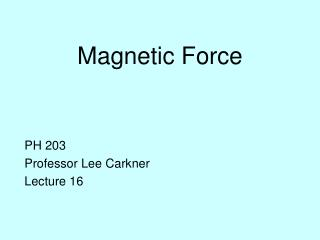DownloadDownload PresentationMagnetic Force

# Magnetic Force

Download Presentation## Magnetic Force

- - - - - - - - - - - - - - - - - - - - - - - - - - - E N D - - - - - - - - - - - - - - - - - - - - - - - - - - -
##### Presentation Transcript

1. Magnetic Force PH 203 Professor Lee Carkner Lecture 16

2. Charge Carriers Imaging a current flowing from top to bottom in a wire, with a magnetic field pointing “in” If the charge carriers are negative (moving to the top), the magnetic field will also deflect them to the right

3. The Hall Effect If it is high the carriers are positive Since a voltmeter shows the low potential is on the right, the electron is negative

4. Hall Quantified Electrons are now longer deflected and the potential across the strip is constant but the velocity is the drift speed of the electrons v = i/neA n = Bi/eAE Since the potential V = Ed and the thickness of the strip (lower case “ell”), l = A/d n = Bi/Vle

5. Electric and Magnetic Force • For a uniform field, electric force vector does not change • Electric fields accelerate particles, magnetic fields deflect particles

6. Particle Motion • A particle moving freely in a magnetic field will have one of three paths, depending on q • Straight line • When q = • Circle • When q = • Helix • When • This assumes a uniform field that the particle does not escape from

7. Circular Motion

8. Circular Motion • This will change the direction of v, and change the direction of F towards more bending • How big is the circle? • Magnetic force is F = • Centripetal force is F = • We can combine to get r = mv/qB • Radius of orbit of charged particle in a uniform magnetic field

9. Circle Properties • Circle radius is inversely proportional to q and B • r is directly proportional to v and m • Can use this idea to make mass spectrometer • Send mixed atoms through the B field and they will come out separated by mass

10. Helical Motion • Charged particles will spiral around magnetic field lines • If the field has the right geometry, the particles can become trapped • Since particles rarely encounter a field at exactly 0 or 90 degrees, such motion is very common • Examples: • Gyrosynchrotron radio emission from planets and stars

11. Helical Motion

12. Magnetic Field and Current • We know that i = q/t and v = L/t (where L is the length of the wire) • So qv = iL, thus: F = BiL sin f • We can use the right hand rule to get the direction of the force • Use the direction of the current instead of v

13. Force on a Wire

14. Force on a Loop of Wire • Consider a loop of wire placed so that it is lined up with a magnetic field • Two sides will have forces at right angles to the loop, but in opposite directions • The loop will experience a torque

15. Loop of Current

16. Torque on Loop • Since q = 90 and L = h, F = Bih • The torque is the force times the moment arm (distance to the center), which is w/2 • but hw is the area of the loop, A t = iBA t = iBA sin q

17. Torque on Loop

18. General Loops t = iBAN sin q • The torque is maximum when the loop is aligned with the field and zero when the field is at right angles to the loop (field goes straight through loop) • If you reverse the direction of the current at just the right time you can get the coil to spin • Can harness the spin to do work

19. Next Time • Read 29.1-29.4 • Problems: Ch 28, P: 22, 36, 67, Ch 29, P: 1, 27 • Test 2 next Friday

20. A beam of electrons is pointing right at you. What direction would a magnetic field have to have to produce the maximum deflection in the right direction? • Right • Left • Up • Down • Right at you

21. A beam of electrons is pointing right at you. What direction would a magnetic field have to have to produce the maximum deflection in the up direction? • Right • Left • Up • Down • Right at you

22. A beam of electrons is pointing right at you. What direction would a magnetic field have to have to produce no deflection? • Right • Left • Up • Down • Right at you